Examples

Chapter 16 Class 8 Playing with Numbers
Serial order wise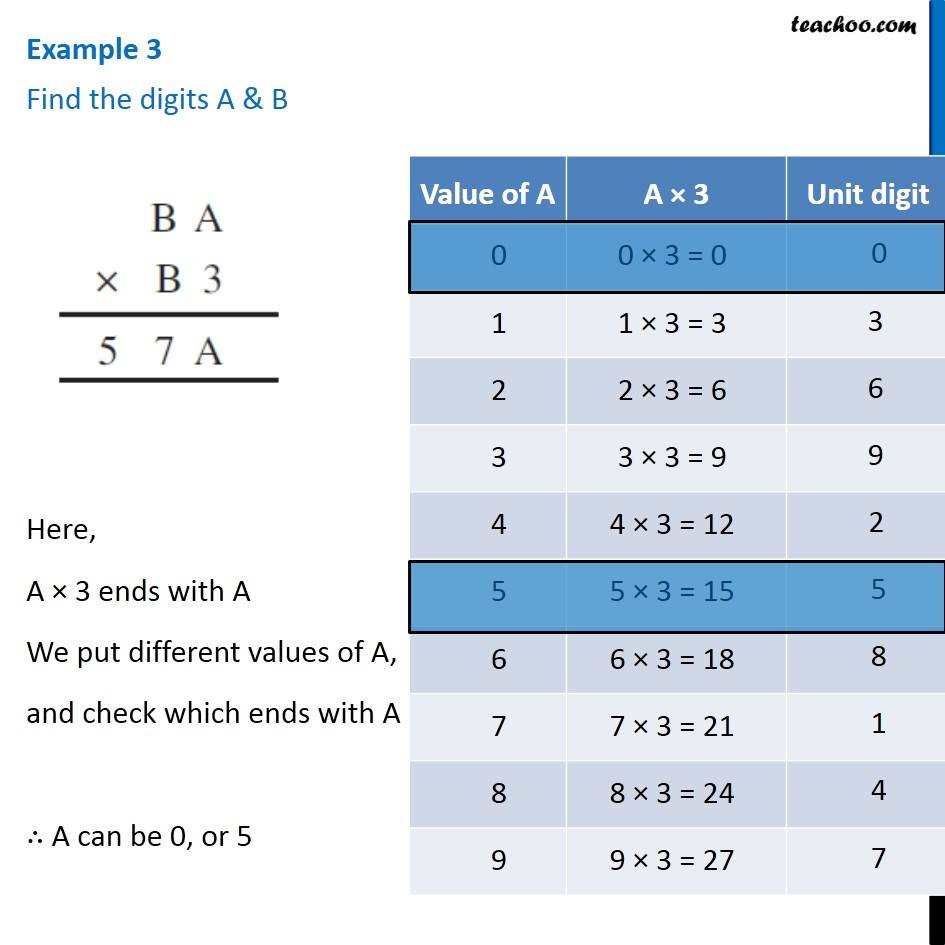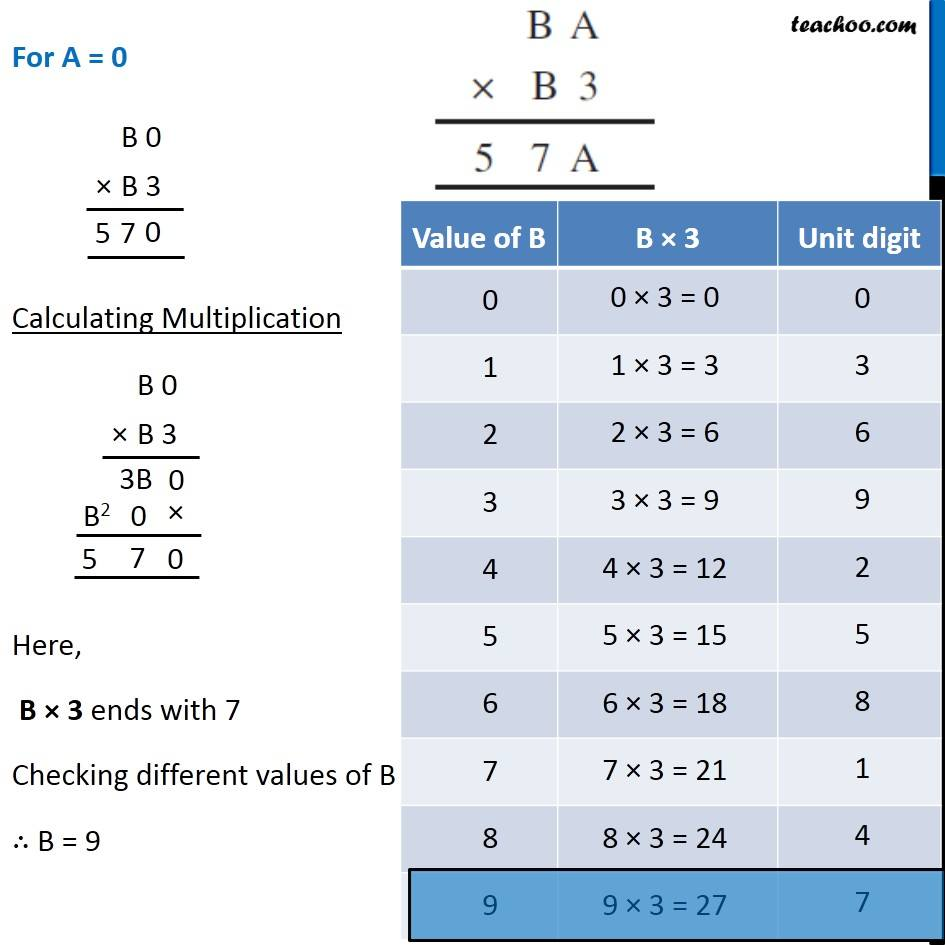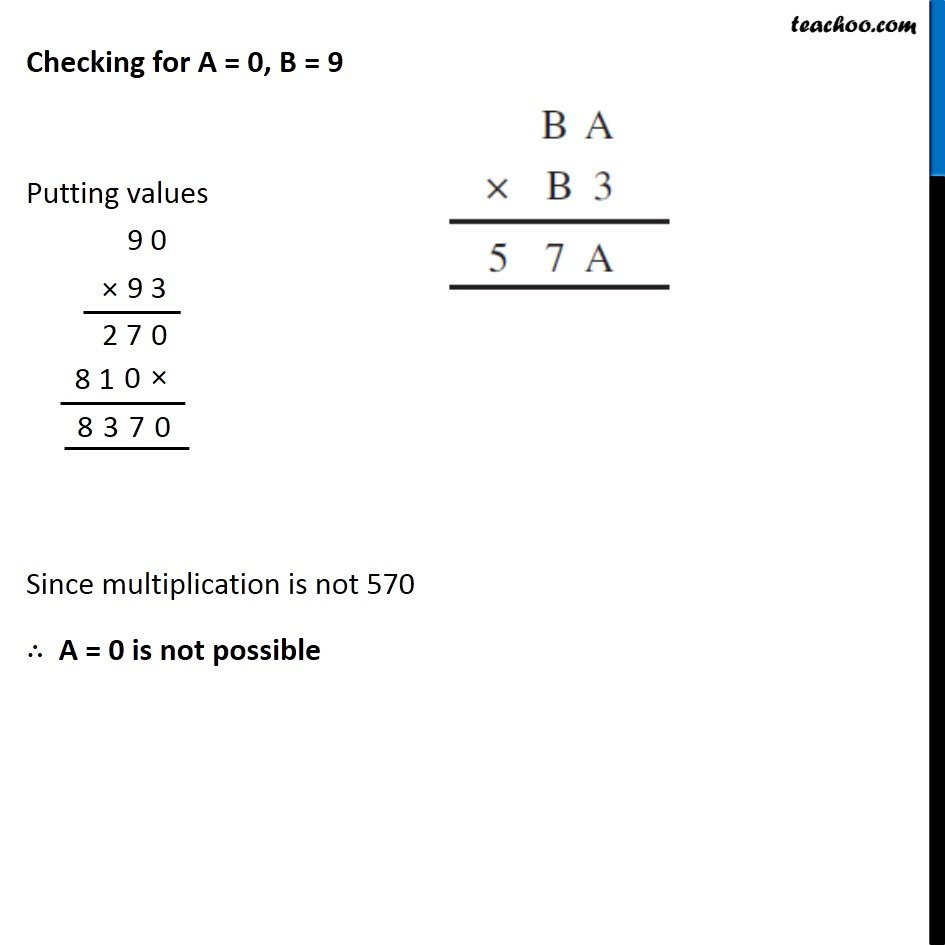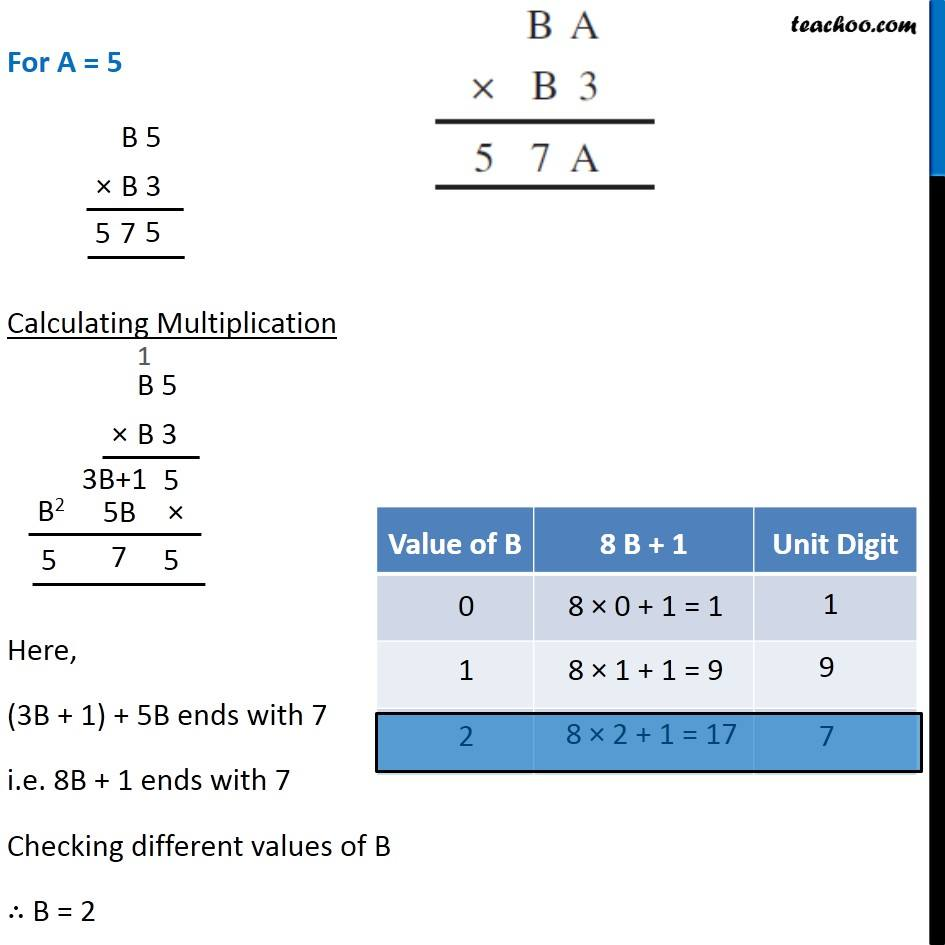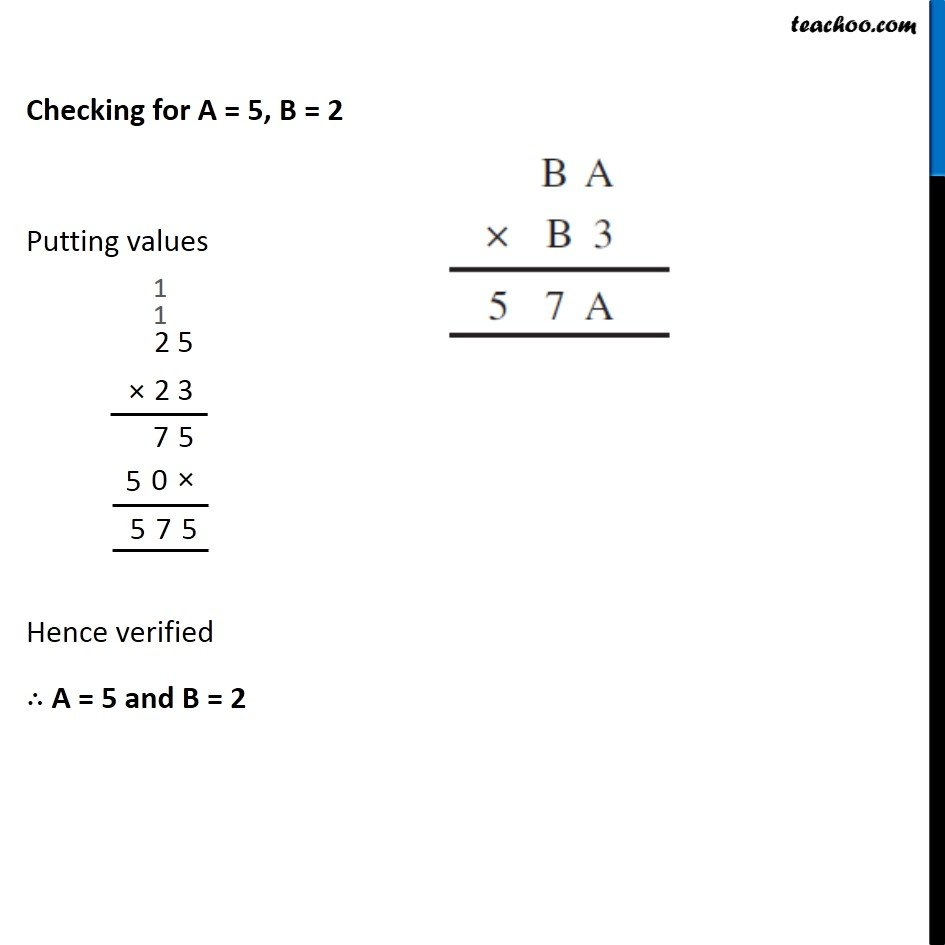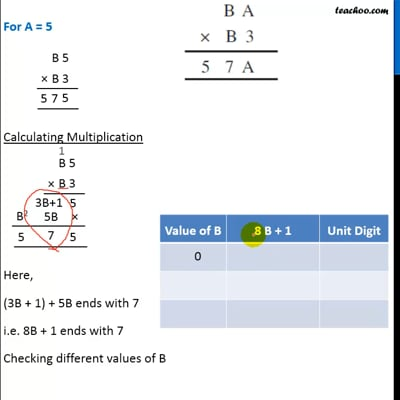This video is only available for Teachoo black users

Introducing your new favourite teacher - Teachoo Black, at only ₹83 per month

### Transcript

Example 3 Find the digits A & B Here, A × 3 ends with A We put different values of A, and check which ends with A ∴ A can be 0, or 5 Checking for A = 0, B = 9 Putting values Since multiplication is not 570 ∴ A = 0 is not possible Checking for A = 0, B = 9 Putting values Since multiplication is not 570 ∴ A = 0 is not possible For A = 5 Calculating Multiplication Here, (3B + 1) + 5B ends with 7 i.e. 8B + 1 ends with 7 Checking different values of B ∴ B = 2 Checking for A = 5, B = 2 Putting values Hence verified ∴ A = 5 and B = 2